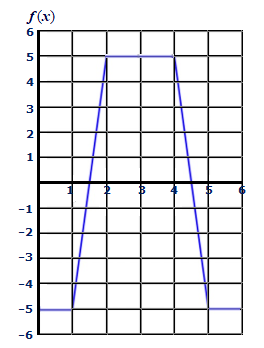# Let A(x) = \int_0^x f(t) dt and B(x) = \int_2^x f(t) dt, with f(x) as in the figure below. Find...

## Question:

Let {eq}A(x) = \int_0^x f(t)\,dt {/eq} and {eq}B(x) = \int_2^x f(t)\,dt {/eq}, with {eq}f(x) {/eq} as in the figure below.

Find formulas for {eq}A(x) {/eq} and {eq}B(x) {/eq} valid on {eq}~[2,4] {/eq}.## Finding formulas :

In this problem we are asked to find the formulae of A(x) , B(x).

Before proceeding further we have to know a basic concept , that is geometric interpretation of integral.

Let f(x) be a function then {eq}\int f(x) \; dx {/eq} represents the area under the curve f(x).

## Answer and Explanation:

In the interval [2,4] f(t) is a constant function , it's equation is f(t) = 5.

{eq}\begin{align*} A(x) &= \int_0^x f(t)\,dt \\ &= \int_0^x 5 \,dt\\ &= 5 \left [ t \right ]_0^x \\ &= 5 x \\ B(x) &= \int_2^x f(t)\,dt \\ &= \int_2^x 5 \,dt \\ &= 5 \left [ t \right ]_2^x \\ &= 5 \cdot x - 10 \end{align*} {/eq}